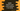# Kotlin program to find the sum of all numbers of an array## Introduction :

Kotlin program to find the sum of all numbers in an array. One number array is given and we need to find out the sum of its numbers.

### Method 1: Using sum() :

Array in kotlin provides one method called sum that returns the sum of all elements of an array. Just call this method sum() to an array :

``````fun main() {
val givenArray = intArrayOf(1,2,3,4,5,6,7,8,9)

println(givenArray.sum())
}``````

It prints 45.

### Method 2: Using fold :

We can also fold the array elements to find the sum of all values :

``````fun main() {
val givenArray = intArrayOf(1,2,3,4,5,6,7,8,9)

println(givenArray.fold(0){acc, e -> acc + e})
}``````

The accumulator acc adds the values of all elements of the array.

### Method 3: Using forEach :

Iterate through the array and add each number to a sum variable :

``````fun main() {
val givenArray = intArrayOf(1, 2, 3, 4, 5, 6, 7, 8, 9)

var sum = 0
givenArray.forEach { sum += it }

println(sum)
}``````

You can also use one normal for loop.

### Method 4: Using reduce :

reduce works like fold. It accumulates all values starting from the first element from left to right :

``````fun main() {
val givenArray = intArrayOf(1, 2, 3, 4, 5, 6, 7, 8, 9)

println(givenArray.reduce { sum, e -> sum + e })
}``````

It will print the sum.

### Example of all methods :

``````fun main() {
val givenArray = intArrayOf(1, 2, 3, 4, 5, 6, 7, 8, 9)

println(givenArray.sum())
println(givenArray.fold(0){acc, e -> acc + e})

var sum = 0
givenArray.forEach { sum += it }
println(sum)

println(givenArray.reduce { s, e -> s + e })
}``````